# Python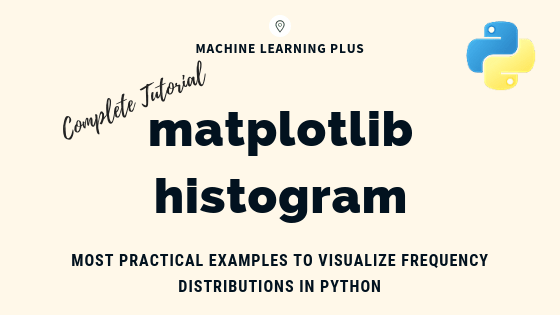## Matplotlib Histogram – How to Visualize Distributions in Python

Matplotlib histogram is used to visualize the frequency distribution of numeric array by splitting it to small equal-sized bins. In this article, we explore practical techniques that are extremely useful in your initial data analysis and plotting. Content What is a histogram? How to plot a basic histogram in python? Histogram grouped by categories in …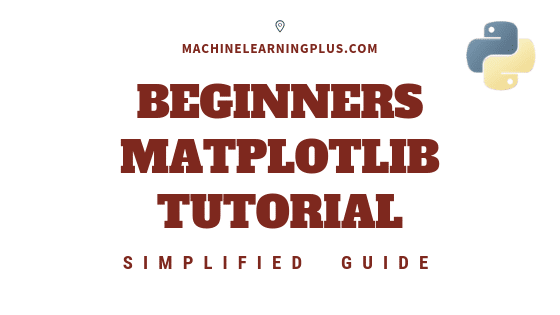## Matplotlib Tutorial – A Complete Guide to Python Plot with Examples

Until next time Until next time This tutorial explains matplotlib’s way of making plots in simplified parts so you gain the knowledge and a clear understanding of how to build and modify full featured matplotlib plots. 1. Introduction Matplotlib is the most popular plotting library in python. Using matplotlib, you can create pretty much any …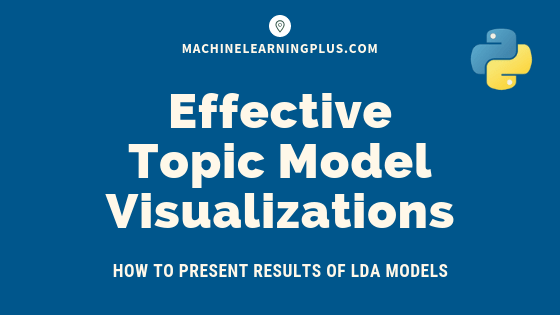## Topic modeling visualization – How to present the results of LDA models?

In this post, we discuss techniques to visualize the output and results from topic model (LDA) based on the gensim package. Topic modeling visualization – How to present the results of LDA models? Contents Introduction Import NewsGroups Dataset Tokenize Sentences and Clean Build the Bigram, Trigram Models and Lemmatize Build the Topic Model Presenting the …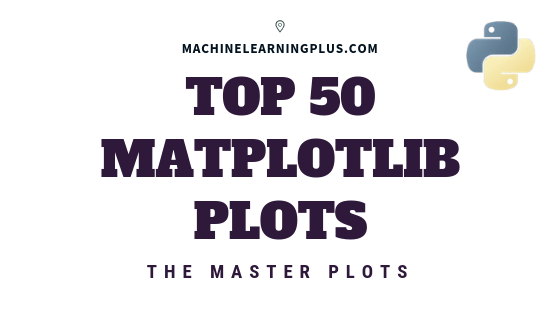## Top 50 matplotlib Visualizations – The Master Plots (with full python code)

A compilation of the Top 50 matplotlib plots most useful in data analysis and visualization. This list lets you choose what visualization to show for what situation using python’s matplotlib and seaborn library. Introduction The charts are grouped based on the 7 different purposes of your visualization objective. For example, if you want to picturize …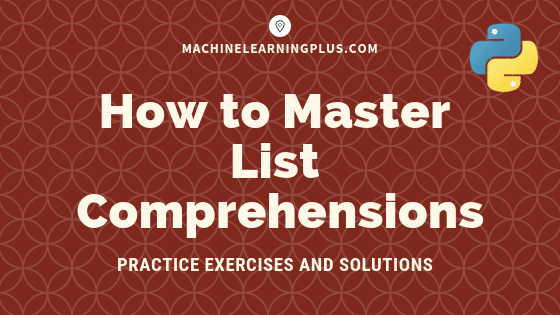## List Comprehensions in Python – My Simplified Guide

List comprehensions is a pythonic way of expressing a ‘For Loop’ that appends to a list in a single line of code. It is an intuitive, easy-to-read and a very convenient way of creating lists. This is a beginner friendly post for those who know how to write for-loops in python but don’t quite understand …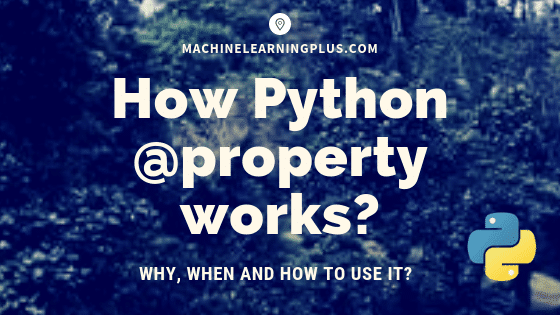## Python @Property Explained – How to Use and When? (Full Examples)

A python @property decorator lets a method to be accessed as an attribute instead of as a method with a ‘()’. Today, you will gain an understanding of when it is really needed, in what situations you can use it and how to actually use it. Contents 1. Introduction2. What does @property do?3. When to …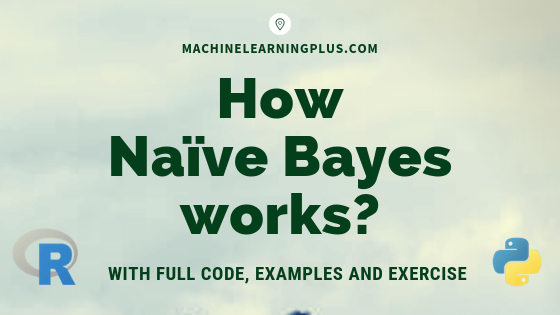## How Naive Bayes Algorithm Works? (with example and full code)

Naive Bayes is a probabilistic machine learning algorithm based on the Bayes Theorem, used in a wide variety of classification tasks. In this post, you will gain a clear and complete understanding of the Naive Bayes algorithm and all necessary concepts so that there is no room for doubts or gap in understanding. Contents 1. …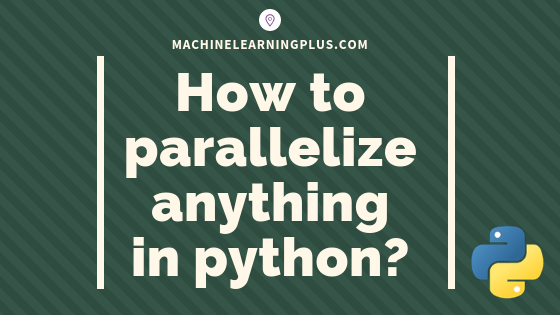## Parallel Processing in Python – A Practical Guide with Examples

Parallel processing is a mode of operation where the task is executed simultaneously in multiple processors in the same computer. It is meant to reduce the overall processing time. In this tutorial, you’ll understand the procedure to parallelize any typical logic using python’s multiprocessing module. 1. Introduction Parallel processing is a mode of operation where …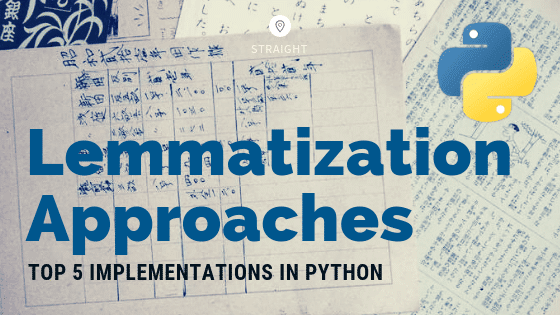## Lemmatization Approaches with Examples in Python

Lemmatization is the process of converting a word to its base form. The difference between stemming and lemmatization is, lemmatization considers the context and converts the word to its meaningful base form, whereas stemming just removes the last few characters, often leading to incorrect meanings and spelling errors. Comparing Lemmatization Approaches in Python. Photo by …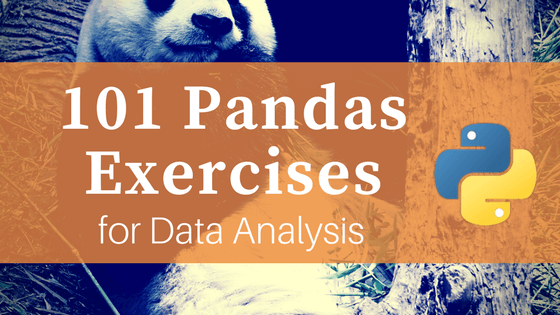## 101 Pandas Exercises for Data Analysis

101 python pandas exercises are designed to challenge your logical muscle and to help internalize data manipulation with python’s favorite package for data analysis. The questions are of 3 levels of difficulties with L1 being the easiest to L3 being the hardest. 101 Pandas Exercises. Photo by Chester Ho. You might also like to practice …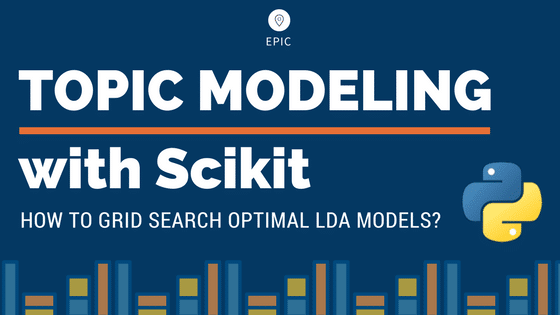## LDA in Python – How to grid search best topic models?

Python’s Scikit Learn provides a convenient interface for topic modeling using algorithms like Latent Dirichlet allocation(LDA), LSI and Non-Negative Matrix Factorization. In this tutorial, you will learn how to build the best possible LDA topic model and explore how to showcase the outputs as meaningful results. Contents 1. Introduction 2. Load the packages 3. Import …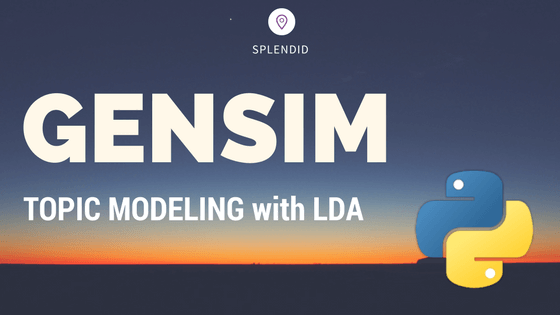## Topic Modeling with Gensim (Python)

Topic Modeling is a technique to extract the hidden topics from large volumes of text. Latent Dirichlet Allocation(LDA) is a popular algorithm for topic modeling with excellent implementations in the Python’s Gensim package. The challenge, however, is how to extract good quality of topics that are clear, segregated and meaningful. This depends heavily on the …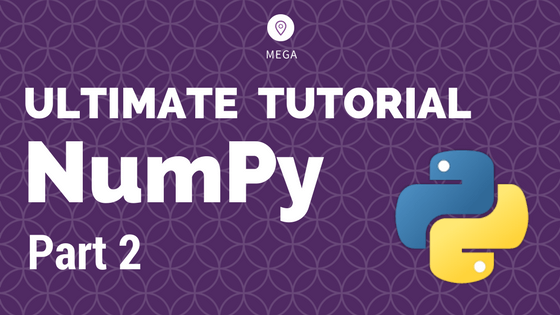## Numpy Tutorial Part 2 – Vital Functions for Data Analysis

Numpy is the core package for data analysis and scientific computing in python. This is part 2 of a mega numpy tutorial. In this part, I go into the details of the advanced features of numpy that are essential for data analysis and manipulations. Introduction How to get index locations that satisfy a given condition …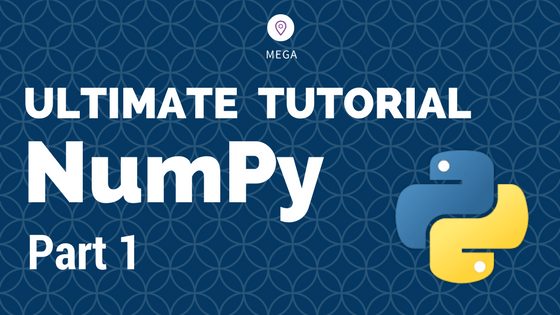## Numpy Tutorial – Your first numpy guide to build python coding foundations

This is part 1 of the numpy tutorial covering all the core aspects of performing data manipulation and analysis with numpy’s ndarrays. Numpy is the most basic and a powerful package for scientific computing and data manipulation in python. Numpy Tutorial Part 1: Introduction to Arrays. Photo by Bryce Canyon. Also Read: Numpy Tutorial – …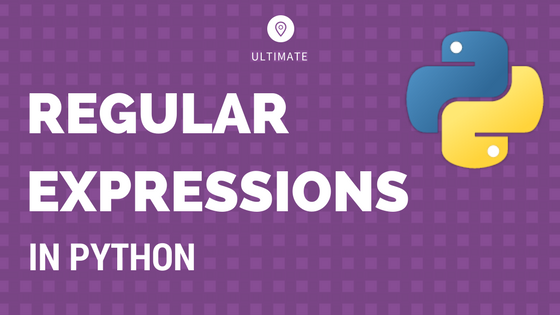## Python Regular Expressions Tutorial and Examples: A Simplified Guide

Regular expressions, also called regex, is a syntax or rather a language to search, extract and manipulate specific string patterns from a larger text. It is widely used in projects that involve text validation, NLP and text mining Regular Expressions in Python: A Simplified Tutorial. Photo by Sarah Crutchfield. Contents 1. Introduction to regular expressions …Course Preview

## Machine Learning A-Z™: Hands-On Python & R In Data Science

### Free Sample Videos:#### Machine Learning A-Z™: Hands-On Python & R In Data Science#### Machine Learning A-Z™: Hands-On Python & R In Data Science#### Machine Learning A-Z™: Hands-On Python & R In Data Science#### Machine Learning A-Z™: Hands-On Python & R In Data Science